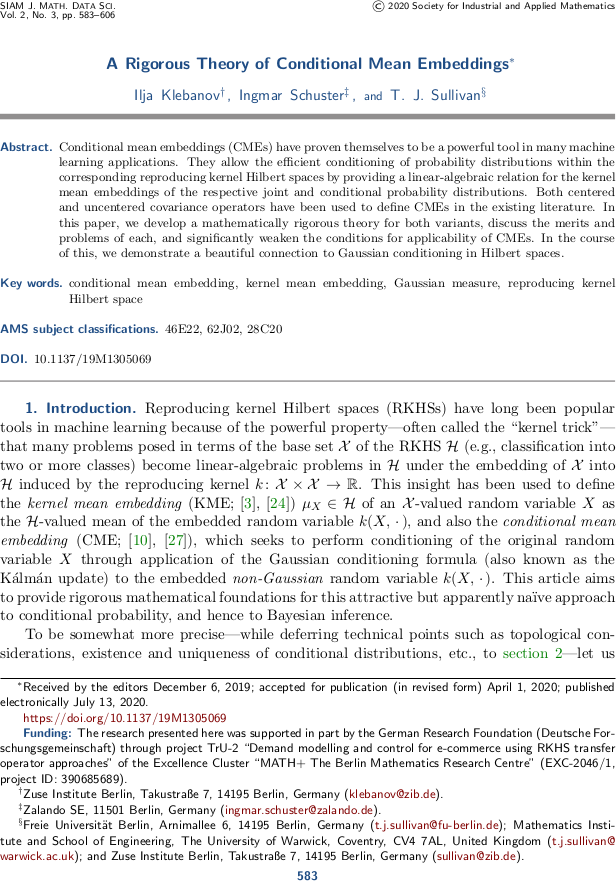# Tim Sullivan### A rigorous theory of conditional mean embeddings in SIMODS

The article “A rigorous theory of conditional mean embeddings” by Ilja Klebanov, Ingmar Schuster, and myself has just appeared online in the SIAM Journal on Mathematics of Data Science. In this work we take a close mathematical look at the method of conditional mean embedding. In this approach to non-parametric inference, a random variable $$Y \sim \mathbb{P}_{Y}$$ in a set $$\mathcal{Y}$$ is represented by its kernel mean embedding, the reproducing kernel Hilbert space element

$$\displaystyle \mu_{Y} = \int_{\mathcal{Y}} \psi(y) \, \mathrm{d} \mathbb{P}_{Y} (y) \in \mathcal{G},$$

and conditioning with respect to an observation $$x$$ of a related random variable $$X \sim \mathbb{P}_{X}$$ in a set $$\mathcal{X}$$ with RKHS $$\mathcal{H}$$ is performed using the Woodbury formula

$$\displaystyle \mu_{Y|X = x} = \mu_Y + (C_{XX}^{\dagger} C_{XY})^\ast \, (\varphi(x) - \mu_X) .$$

Here $$\psi \colon \mathcal{Y} \to \mathcal{G}$$ and $$\varphi \colon \mathcal{X} \to \mathcal{H}$$ are the canonical feature maps and the $$C$$'s denote the appropriate centred (cross-)covariance operators of the embedded random variables $$\psi(Y)$$ in $$\mathcal{G}$$ and $$\varphi(X)$$ in $$\mathcal{H}$$.

Our article aims to provide rigorous mathematical foundations for this attractive but apparently naïve approach to conditional probability, and hence to Bayesian inference.

I. Klebanov, I. Schuster, and T. J. Sullivan. “A rigorous theory of conditional mean embeddings.” SIAM Journal on Mathematics of Data Science 2(3):583–606, 2020. doi:10.1137/19M1305069

Abstract. Conditional mean embeddings (CMEs) have proven themselves to be a powerful tool in many machine learning applications. They allow the efficient conditioning of probability distributions within the corresponding reproducing kernel Hilbert spaces by providing a linear-algebraic relation for the kernel mean embeddings of the respective joint and conditional probability distributions. Both centered and uncentered covariance operators have been used to define CMEs in the existing literature. In this paper, we develop a mathematically rigorous theory for both variants, discuss the merits and problems of each, and significantly weaken the conditions for applicability of CMEs. In the course of this, we demonstrate a beautiful connection to Gaussian conditioning in Hilbert spaces.

Published on Wednesday 15 July 2020 at 08:00 UTC #publication #simods #mathplus #tru2 #rkhs #mean-embedding #klebanov #schuster